## Exercises

1. Consider the obstacle region, (7.1), in the state space for time-varying motion planning.
1. To ensure that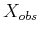is polyhedral, what kind of paths should be allowed? Show how the model primitives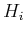that define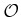are expressed in general, using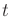as a parameter.
2. Repeat the exercise, but for ensuring thatis semi-algebraic.
2. Propose a way to adapt the sampling-based roadmap algorithm of Section 5.6 to solve the problem of time-varying motion planning with bounded speed.
3. Develop an efficient algorithm for computing the obstacle region for two translating polygonal robots that each follow a linear path.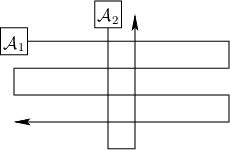4. Sketch the coordination space for the two robots moving along the fixed paths shown in Figure 7.43.
5. Suppose there are two robots, and each moves on its own roadmap of three paths. The paths in each roadmap are arranged end-to-end in a triangle.
1. Characterize the fixed-roadmap coordination space that results, including a description of its topology.
2. Now suppose there arerobots, each on a triangular roadmap, and characterize the fixed-roadmap coordination space.
6. Consider the state space obtained as the Cartesian product of the C-spaces ofidentical robots. Suppose that each robot is labeled with a unique integer. Show thatcan be partitioned nicely into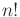regions in whichappears identical and the only difference is the labels (which indicate the particular robots that are in collision).
7. Suppose there are two robots, and each moves on its own roadmap of three paths. The paths in one roadmap are arranged end-to-end in a triangle, and the paths in the other are arranged as a Y. Characterize the fixed-roadmap coordination space that results, including a description of its topology.
8. Design an efficient algorithm that takes as input a graph representation of the connectivity of a linkage and computes an active-passive decomposition. Assume that all links are revolute. The algorithm should work for either 2D or 3D linkages (the dimension is also an input). Determine the asymptotic running time of your algorithm.
9. Consider the problem of coordinating the motion of two robots that move along precomputed paths but in the presence of predictable moving obstacles. Develop a planning algorithm for this problem.
10. Consider a manipulator in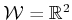made of four links connected in a chain by revolute joints. There is unit distance between the joints, and the first joint is attached at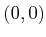in. Suppose that the end of the last link, which is position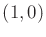in its body frame, is held at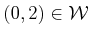.
1. Use kinematics expressions to express the closure constraints for a configuration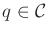.
2. Convert the closure constraints into polynomial form.
3. Use differentiation to determine the constraints on the allowable velocities that maintain closure at a configuration.

Implementations

11. Implement the vertical decomposition algorithm to solve the path-tuning problem, as shown in Figure 7.5.
12. Use grid-based sampling and a search algorithm to compute collision-free motions of three robots moving along predetermined paths.
13. Under the conditions of Exercise 12, compute Pareto-optimal coordination strategies that optimize the time (number of stages) that each robot takes to reach its goal. Design a wavefront propagation algorithm that keeps track of the complete (ignoring equivalent strategies) set of minimal Pareto-optimal coordination strategies at each reached state. Avoid storing entire plans at each discretized state.
14. To gain an appreciation of the difficulties of planning for closed kinematic chains, try motion planning for a point on a torus among obstacles using only the implicit torus constraint given by (6.40). To simplify collision detection, the obstacles can be a collection of balls in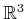that intersect the torus. Adapt a sampling-based planning technique, such as the bidirectional RRT, to traverse the torus and solve planning problems.
15. Implement the spanning-tree coverage planning algorithm of Section 7.6.
16. Develop an RRT-based planning algorithm that causes the robot to chase an unpredictable moving target in a planar environment that contains obstacles. The algorithm should run quickly enough so that replanning can occur during execution. The robot should execute the first part of the most recently computed path while simultaneously computing a better plan for the next time increment.
17. Modify Exercise 16 so that the robot assumes the target follows a predictable, constant-velocity trajectory until some deviation is observed.
18. Show how to handle unexpected obstacles by using a fast enough planning algorithm. For simplicity, suppose the robot is a point moving in a polygonal obstacle region. The robot first computes a path and then starts to execute it. If the obstacle region changes, then a new path is computed from the robot's current position. Use vertical decomposition or another algorithm of your choice (provided it is fast enough). The user should be able to interactively place or move obstacles during plan execution.
19. Use the manipulation planning framework of Section 7.3.2 to develop an algorithm that solves the famous Towers of Hanoi problem by a robot that carries the rings . For simplicity, suppose a polygonal robot moves polygonal parts inand rotation is not allowed. Make three pegs, and initially place all parts on one peg, sorted from largest to smallest. The goal is to move all of the parts to another peg while preserving the sorting.
20. Use grid-based approximation to solve optimal planning problems for a point robot in the plane. Experiment with using different neighborhoods and metrics. Characterize the combinations under which good and bad approximations are obtained.

Steven M LaValle 2020-08-14